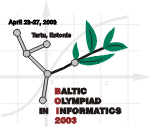Baltic Olympiad in Informatics
April 23-27, 2003, Tartu, Estonia

# Gems

The Gem-Toys Company asked you to solve the following problem.

You are given a connected acyclic graph, i.e. a set of vertices connected by edges in such a way that from each vertex you can reach all the other vertices by traversing the edges, and it does not contain a loop.

The Gem-Toys Company is going to produce jewelry models of such graphs. Vertices will be made of gems and edges will be made of gold string. It is required that adjacent vertices are made of different kinds of gems. For each positive integer p there is exactly one kind of gems with the price p.

Your task is to write a program computing the minimal total price of the gems needed to make the model.

## Input data

The first line of the input file GEMS.IN contains one positive integer N (1 ≤ N ≤ 10 000), the number of vertices. The vertices are numbered from 1 to N. The following N-1 lines describe the edges, one per line. Each of these lines contains a pair of integers A and B separated by a space (1 ≤ A, B ≤ N, A ≠ B). Such a pair represents an edge connecting vertices A and B.

## Output data

The first and only line of the output file GEMS.OUT must contain one integer: the minimal total price of the gems needed to make the model.

## Sample

 GEMS.IN GEMS.OUT 8 1 2 3 1 1 4 5 6 1 5 5 7 5 8 11